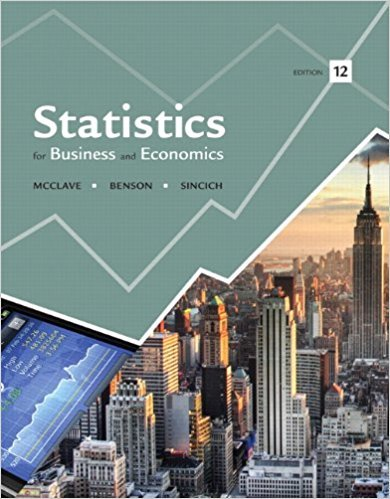# Suppose you fit the first-order modely = ? 0 + ? 1 x 1 + ?## Problem 7E Chapter 12

Statistics for Business and Economics | 12th Edition

• 2901 Step-by-step solutions solved by professors and subject experts
• Get 24/7 help from StudySoup virtual teaching assistantsStatistics for Business and Economics | 12th Edition

4 5 0 330 Reviews
10
2
Problem 7E

Suppose you fit the first-order model

y = β 0 + β 1 x 1 + β 2 x 2 + β 3 x 3 + β 4 x 4 + β 5 x 5 + ε

to n = 30 data points and obtain

SSE = .33 R2 = .92

a. Do the values of SSE and R2 suggest that the model provides a good fit to the data? Explain.

b. Is the model of any use in predicting y? Test the null hypothesis H0: β1 = β2 = β3 = β4 = β5 = 0 against the alternative hypothesis Ha: At least one of the parameters β1, β2, …., β5 is nonzero. Use α = .05.

Step-by-Step Solution:
Step 2 of 3
Step 3 of 3

##### ISBN: 9780321826237

Unlock Textbook Solution

Suppose you fit the first-order modely = ? 0 + ? 1 x 1 + ?

×
Get Full Access to Statistics For Business And Economics - 12th Edition - Chapter 12 - Problem 7e

Get Full Access to Statistics For Business And Economics - 12th Edition - Chapter 12 - Problem 7e

I don't want to reset my password

Need help? Contact support

Need an Account? Is not associated with an account
We're here to help